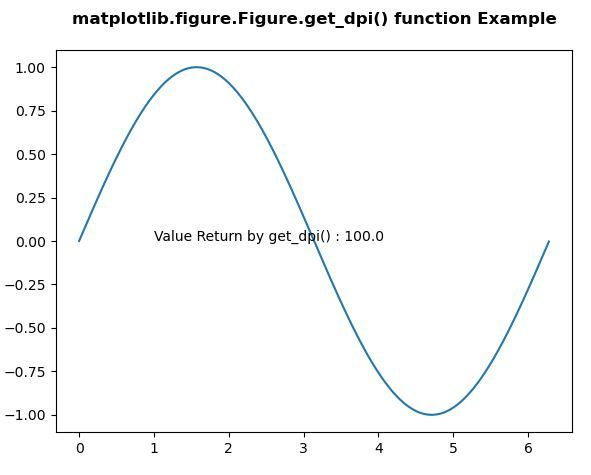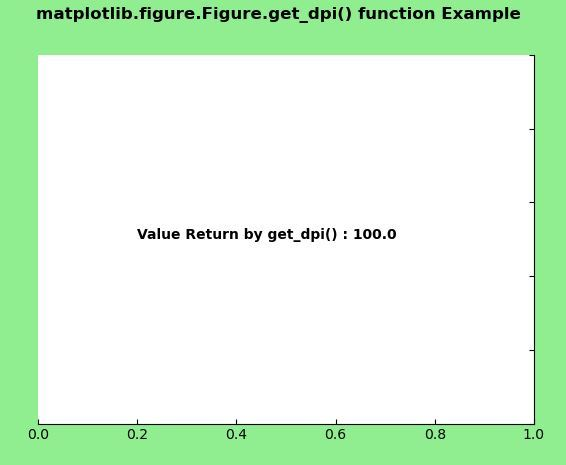Skip to content
Related Articles
Matplotlib.figure.Figure.get_dpi() in Python
• Last Updated : 30 Apr, 2020

Matplotlib is a library in Python and it is numerical – mathematical extension for NumPy library. The figure module provides the top-level Artist, the Figure, which contains all the plot elements. This module is used to control the default spacing of the subplots and top level container for all plot elements.

## matplotlib.figure.Figure.get_dpi() method

The get_dpi() method of figure module of matplotlib library is used to get the resolution in dots per inch as a float.

Syntax: get_dpi(self)

Parameters: This method does not accept any parameters.

Returns: This method return the resolution in dots per inch as a float.

Below examples illustrate the matplotlib.figure.Figure.get_dpi() function in matplotlib.figure:

Example 1:

 `# Implementation of matplotlib function ``import` `matplotlib.pyplot as plt ``from` `matplotlib.figure ``import` `Figure``import` `numpy as np ``    ` `fig ``=` `plt.figure(figsize ``=``(``5``, ``4``)) ``    ` `ax ``=` `fig.add_axes([``0.1``, ``0.1``, ``0.8``, ``0.8``])``  ` `xx ``=` `np.arange(``0``, ``2` `*` `np.pi, ``0.01``) ``ax.plot(xx, np.sin(xx)) `` ` `w ``=` `fig.get_dpi()``ax.text(``1``, ``0``, ``"Value Return by get_dpi() : "``        ``+``str``(w))``   ` `fig.canvas.draw()``fig.suptitle('matplotlib.figure.Figure.get_dpi() function \``Example', fontweight ``=``"bold"``) `` ` `plt.show()`

Output:Example 2:

 `# Implementation of matplotlib function ``import` `matplotlib.pyplot as plt ``from` `matplotlib.figure ``import` `Figure``from` `mpl_toolkits.axisartist.axislines ``import` `Subplot ``import` `numpy as np ``   ` ` ` `fig ``=` `plt.figure(facecolor ``=``"lightgreen"``) ``    ` `ax ``=` `Subplot(fig, ``111``) ``fig.add_subplot(ax) ``    ` `ax.axis[``"left"``].set_visible(``False``) ``ax.axis[``"top"``].set_visible(``False``)`` ` `w ``=` `fig.get_dpi()``ax.text(``0.2``, ``0.5``, ``"Value Return by get_dpi() : "``        ``+``str``(w),``        ``fontweight ``=``"bold"``)``   ` `fig.canvas.draw()``fig.suptitle('matplotlib.figure.Figure.get_dpi()\`` ``function Example', fontweight ``=``"bold"``) `` ` `plt.show()`

Output:Attention geek! Strengthen your foundations with the Python Programming Foundation Course and learn the basics.

To begin with, your interview preparations Enhance your Data Structures concepts with the Python DS Course. And to begin with your Machine Learning Journey, join the Machine Learning – Basic Level Course

My Personal Notes arrow_drop_up